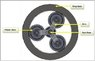# Quantify energy on planet gear

• John D
In summary, John D argues that all the energy that goes through a planetary gear set goes through the planet gear and that this energy can be quantified as power with torque and rpm relative to the axis of the planet gear. He says that this power can be calculated using the power on the carrier and the rpm of the planet gear. He also points out that the ratio between the torque on the planet and the torque on the input is always the same.f

#### John D

How can I quantify the energy on the planet gear of a planetary gear set?

My thoughts are:

All the energy that goes through a planetary gear set goes through the planet gear.

This energy can be quantified as power with torque and rpm relative to the axis of the planet gear.

Thanks

John D

quantify the energy on the planet gear
"Gear(s)? Energy? Or torques/loads? Ring or sun stationary?

I was hoping for energy as in power. rpm x torque / 5252 = horse power.
As if it was a gear in a sequential gear train with torque rpm and horsepower. We all chase torque through a gear set using our rules but all the parts have power, energy. I thought.
thanks Bystander,
John D

Please John, can you tell me which are the input (power) and the output (load) wheel in order to know the power flux?

#### Attachments

•image.jpg
22.9 KB · Views: 298
flemon38
Because all the energy that goes through the planet set goes through the planet gear I would think the energy should be calculated the same. For many years we have been using this gear system with one member fixed. Lately we have been using it in power split situations as in CVTs and hybrid systems. You could use the example of power in on the planet carrier (arm) and out on the sun and ring. Even with the sun or ring stopped all power is always going through the planet gear.
Pc = Pp = Pr + Ps Power on the carrier equals the power on the planet equals power on the ring plus the sun.
Thanks for any help,
John D

Please John, can you tell me which are the input (power) and the output (load) wheel in order to know the power flux?

Pc = Pp = Pr + Ps
I'm more inclined to write this as Pcarrier = nPplanet, where "n" is the number of planetaries.

Then John, if N is the torque:

Np.wp=Ns.ws+Nr.wr

and then:

Np/zp=Ns/zs+Nr./zr

Well, I don't know if this was what you were looking for.

Yes
I can calculate the rpm but how do I calculate the torque? Can I just use the power on the carrier and the rpm of the planet gear?
Bystander is right. For purposes of understanding I was using just one planet. The point is the total power is on the planet(s). Power*5252/rpm on the planet?
Thanks guys,
John D

If we have these six variables: N's and w's, and the followings three independent equations:
Np.wp=Ns.ws+Nr.wr
ws=wp.zp/zs
wr=wp.zp/zr

then we need three knows variables in order to get the other three unknows

The unknown appears to be the torque ( Np)
on the planet.

A more realistic example could be, for instance the following: the two outputs could be two brakes so we will know their torques, Ns and Nr, the other date could be the characteristcs curve of the input motor: f(Np,wp)=0. So, from the first equation we could know the power of the motor and, from the characteristics curve Np and wp. From the other two equations, ws and wr

Felmon38,
This is my question? Can I make the statements: The power on the planet is equal to the power on the input. The power on the planet can be expressed in torque and rpm. Ignoring efficiency. A side question would be; is the ratio between Ns and Nr always the same?
Thanks

According with the equations I don't think so.

I agree with you 100%.

If any of the advisors are watching I would expect we will get a disagreement.
There is an equal force on the ring and sun rule that gets in the way.
That is really what I would like to get a conversation started about.
Thanks felmon38,
John D

Sorry John, I've spent this long time to understand your question (this is my fault not yours) well: there is not a rule at all telling that the force on the sun and on the ring are the same (I suppose we are talking about the forces the planet exerts on the ring and on the sun)
Bye